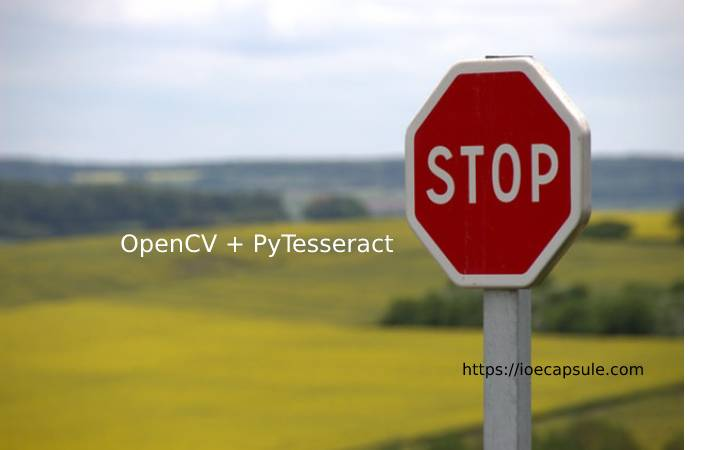### Text Detection Using OpenCV and PyTesseractUncategorized

Recognizing text from an image is easier these days because of the libraries like OpenCV and pytesseract . In this article, I will show you how you can implement simple text recognizer using python.

I am using `python3.6 `. Some required libraries are :

1. OpenCV `sudo pip install opencv`
2. Numpy `sudo pip install numpy`
3. PyTesseract `sudo pip install pytesseract; sudo apt-get install tesseract-ocr`

Now let’s get to the coding part.

1. Create a working folder and name it as you like
2. Create a folder img inside working folder
3. Create a folder temp inside working folder
4. Put some test images in img folder
5. Create a file `detector.py `and write some code
``````
import cv2
import numpy as np
import pytesseract as tes
from PIL import Image
import os

def get_string(path):
#gray conversion
img = cv2.cvtColor(img, cv2.COLOR_BGR2GRAY)

#removing noise
kernel = np.ones((1, 1), np.uint8)
img = cv2.dilate(img, kernel, iterations = 1)
img = cv2.erode(img, kernel, iterations = 1)

#write noise free image
cv2.imwrite("./temp/noise_free.png", img)

#apply threashold

#write again
cv2.imwrite("./temp/thres.png", img)

#run tesseract
result = tes.image_to_string(Image.open("./temp/thres.png"))

#remove created files
os.remove("./temp/thres.png")
os.remove("./temp/noise_free.png")

return result

print("starting recognizing...")
#change name.png to actual image file name
print(get_string("./img/name.png"))``````

That’s it. You are good to go. Now run it using

``python3 detector.py``

And you will see the detected text from image.